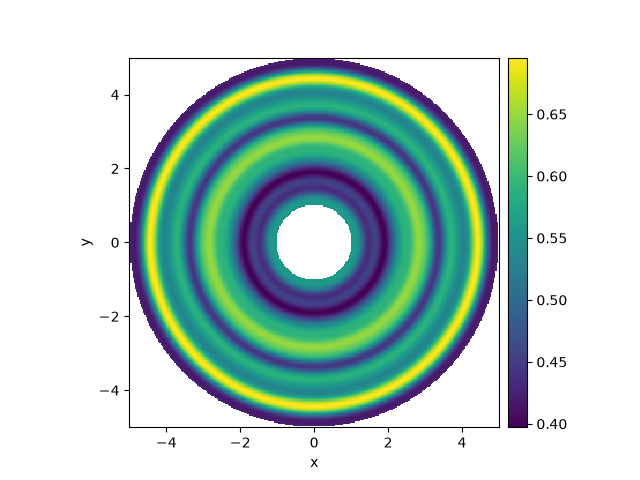# 2.7. Spherically symmetric PDE

This example illustrates how to solve a PDE in a spherically symmetric geometry.```  0%|          | 0/0.1 [00:00<?, ?it/s]
Initializing:   0%|          | 0/0.1 [00:00<?, ?it/s]
0%|          | 0/0.1 [00:02<?, ?it/s]
7%|▋         | 0.007/0.1 [00:02<00:33, 355.03s/it]
35%|███▌      | 0.035/0.1 [00:02<00:04, 71.02s/it]
35%|███▌      | 0.035/0.1 [00:02<00:04, 71.04s/it]
100%|██████████| 0.1/0.1 [00:02<00:00, 24.87s/it]
100%|██████████| 0.1/0.1 [00:02<00:00, 24.87s/it]
```

```from pde import DiffusionPDE, ScalarField, SphericalSymGrid

grid = SphericalSymGrid(radius=[1, 5], shape=128)  # generate grid
state = ScalarField.random_uniform(grid)  # generate initial condition

eq = DiffusionPDE(0.1)  # define the PDE
result = eq.solve(state, t_range=0.1, dt=0.001)

result.plot(kind="image")
```

Total running time of the script: (0 minutes 2.745 seconds)